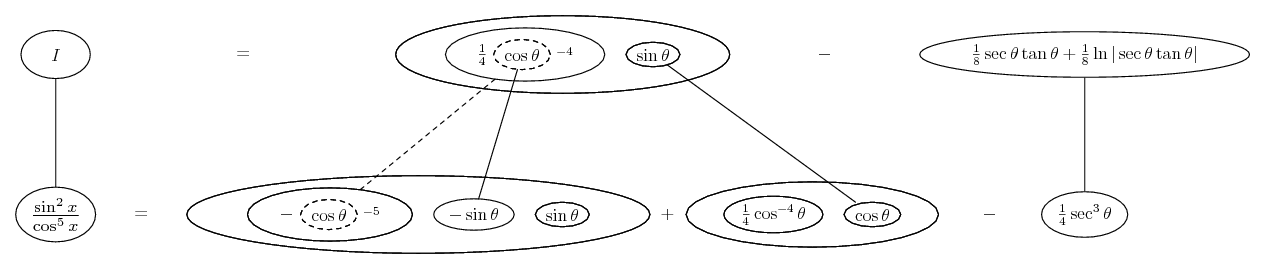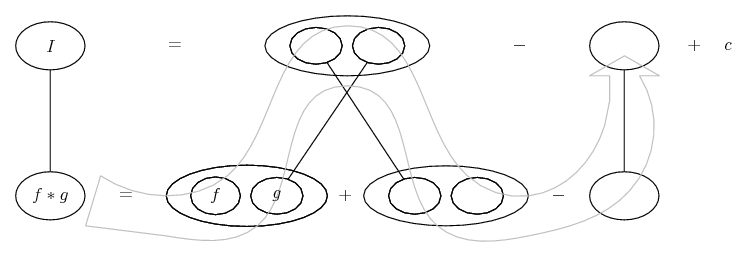# Thread: Solve The Following Trigonometric Integral. (5)

1. ## Solve The Following Trigonometric Integral. (5)

Hello
Here we ge:
$\displaystyle \int tan^2(x) sec^3(x) dx$

I lost2. Hi

The result is horriblehttp://integrals.wolfram.com/index.jsp?expr=tan^2%28x%29+sec^3%28x%29&random=fa lse

3. that's one of the biggest reasons why i don't like much that kind of sites, because they give you an answer that you wouldn't probably expect.

we have $\displaystyle \int{\tan ^{2}(x)\sec ^{3}(x)\,dx}=\int{\frac{\sin ^{2}x}{\cos ^{5}x}\,dx}=\int{\left( \frac{1}{4\cos ^{4}x} \right)'\sin (x)\,dx},$ now integrate by parts and get $\displaystyle \frac{1}{4}\tan (x)\sec ^{3}(x)-\frac{1}{4}\int{\sec ^{3}x\,dx},$ and the remaining integral is quite known, so i think you can solve it.

4.Originally Posted by Krizalidthat's one of the biggest reasons why i don't like much that kind of sites, because they give you an answer that you wouldn't probably expect.

we have $\displaystyle \int{\tan ^{2}(x)\sec ^{3}(x)\,dx}=\int{\frac{\sin ^{2}x}{\cos ^{5}x}\,dx}=\int{\left( \frac{1}{4\cos ^{4}x} \right)'\sin (x)\,dx},$ now integrate by parts and get $\displaystyle \frac{1}{4}\tan (x)\sec ^{3}(x)-\frac{1}{4}\int{\sec ^{3}x\,dx},$ and the remaining integral is quite known, so i think you can solve it.Wow, how did you get the 1/4 from \intsin^2\cos^5) ?

5.Originally Posted by UnsderWow, how did you get the 1/4 from \intsin^2\cos^5) ?
This is called integration by parts.

http://www.mathhelpforum.com/math-he...adult-way.html

6. I understand integration by parts, but I'm not following the sequence from when he has sin^2/cos^5 to when he gets 1/4 in his solution. Sorry for the poor typing, I'm doing this on my phone hehe...can you explain his integration by parts at that point?

7. You are possibly caused to do a double-take by Krizalid's unusual notation for parts, which is explained at Miss's Link. Just in case you are up for further novelties, how about a picture...

Spoiler:... where... is the product rule, and...... the chain rule. Straight continuous lines differentiate downwards (integrate up) with respect to x, and the straight dashed line similarly but with respect to the dashed balloon expression (the inner function of the composite which is subject to the chain rule).

The general drift is...... except we've zoomed in (to the legs-uncrossed version) to show the chain rule which is kind of embedded.

In balloon world, integration by parts is a matter of filling out the product-rule shape usefully but starting in one of the bottom corners instead of (as happens in differentiation) the top one.

This way (as with Krizalid's) you don't need to worry about u du and v dv. (Although choosing legs crossed or uncrossed is to the same purpose.) The shapes can be a bit startling at first, but you might find them relatively straight-forward when you need to differentiate.

_________________________________________

Don't integrate - balloontegrate!

Balloon Calculus; standard integrals, derivatives and methods

Balloon Calculus Drawing with LaTeX and Asymptote!

8. I think I'm being a bit misunderstood.

I know how to do Integration by parts. That part I don't really struggle with, although I'm definetely not perfect at it. What I need to know is how does he get this $\displaystyle \int{\frac{\sin ^{2}x}{\cos ^{5}x}\,dx}$ to $\displaystyle =\int{\left( \frac{1}{4\cos ^{4}x} \right)'\sin (x)\,dx},$

I understand he is using integration by parts, but what exactly is he substituting?

9.Originally Posted by Unsderhow does he get this $\displaystyle \int{\frac{\sin ^{2}x}{\cos ^{5}x}\,dx}$ to $\displaystyle =\int{\left( \frac{1}{4\cos ^{4}x} \right)'\sin (x)\,dx},$
I think you are startled by the absence of u du and v dv, so here they are...

$\displaystyle dv\ =\ \frac{\sin x}{\cos ^{5}x}\ \Rightarrow\ v\ =\ \frac{1}{4}\,\frac{1}{\cos ^{4}x}$

$\displaystyle u\ =\ \sin (x)\ \ \Rightarrow\ du\ = \$ ... etc.

If this works better for you, then stick to it of course!

10. YES TOM! Thanks so much, thats exactly what I needed to see!

#### Search Tags

integral, solve, trigonometric